Select Page

# CBSE MCQ Maths 12 Science Three Dimensional Geometry Solutions in English

CBSE MCQ Maths 12 Science Three Dimensional Geometry Solutions in English to enable students to get Solutions in a narrative video format for the specific question.

Expert Teacher provides CBSE MCQ Maths 12 Science Three Dimensional Geometry Solutions through Video Solutions in English language. This video solution will be useful for students to understand how to write an answer in exam in order to score more marks. This teacher uses a narrative style for a question from Three Dimensional Geometry not only to explain the proper method of answering question, but deriving right answer too.

Please find the question below and view the Solution in a narrative video format.

Question:

Solution Video in English:

## Similar Questions from CBSE, 12th Science, Maths, Three Dimensional Geometry

Question 1 : Find the distance of the plane : 3x - 4y + 12z = 3 from the origin. (View Answer Video)

Question 2 : Find the angle between line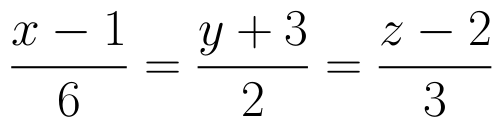and the plane 2x - y + 2z -13 = 0. (View Answer Video)

Question 3 : Find the distance of the point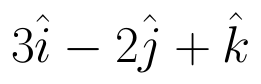from the plane 3x + y - z + 2+0 measured parallel to the line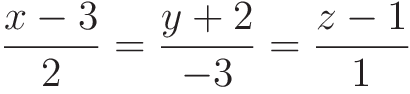Also, find the foot of the perpendicular from the given point upon the given plane. (View Answer Video)

Question 4 : If the equation of a line AB is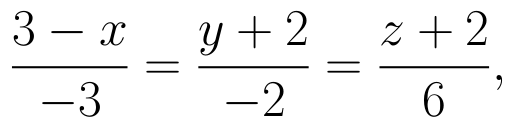then, find the direction cosines of a line parallel to AB. (View Answer Video)

Question 5 : Find the angle between the planes 7x + 2y + 6z = 15 and 3x - y + 10z = 17. (View Answer Video)

### Application of Integrals

Question 1 : Find the area of the smaller region bounded by the ellipse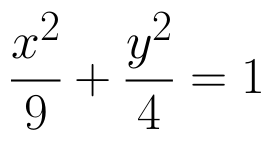and the line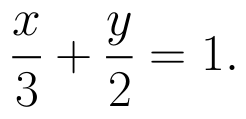(View Answer Video)

Question 2 : Find the area bounded by curves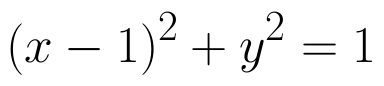and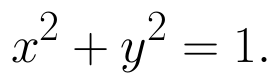(View Answer Video)

Question 3 : Find the area enclosed by the parabola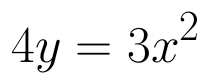and the line 2y = 3x + 12.   (View Answer Video)

Question 4 : Using integration, find the area of the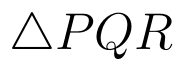co-ordinates whose vertices are P(2,0), Q(4, 5) and R(6,3). (View Answer Video)

Question 5 : Find the area bounded by curves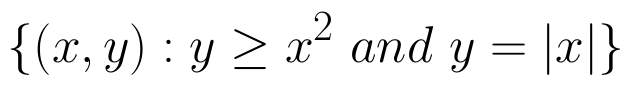. (View Answer Video)

### Differential Equations

Question 1 : Find the particular solution of the differential equation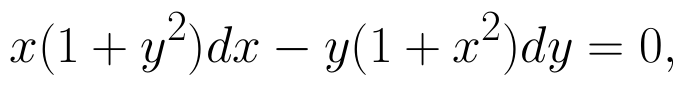given that y = 1 when x = 0.  (View Answer Video)

Question 2 :  Find the particular solution of the differential equation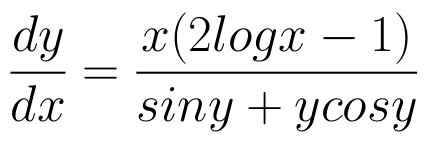given that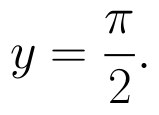When x =1.  (View Answer Video)

Question 3 : Write the sum of the order and degree of the differential equation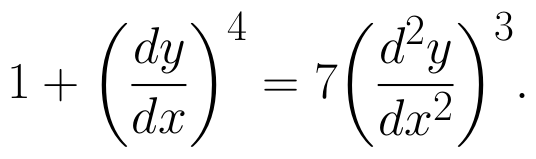(View Answer Video)

Question 4 : Obtain the differential equation of the family of circles passing through the points (a, 0) and (-a, 0). (View Answer Video)

Question 5 : Find the sum of the order and the degree of the following differential equation: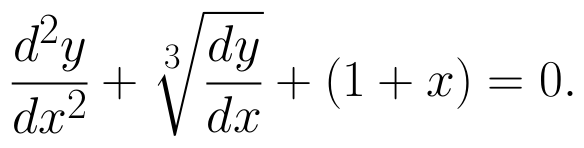(View Answer Video)

### Continuity and Differentiability

Question 1 : Differentiate w.r.t.x the function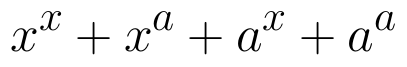, for some fixed a > 0 and x > 0. (View Answer Video)

Question 2 : Differentiate the function w.r.t.x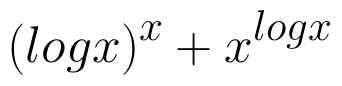. (View Answer Video)

Question 3 : Findfor the function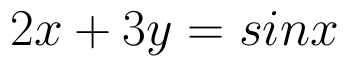. (View Answer Video)

Question 4 :  Find the second order derivative of the function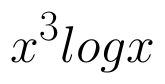. (View Answer Video)

Question 5 : Differentiate the function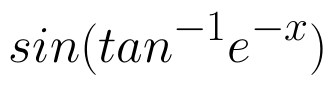with respect to x. (View Answer Video)